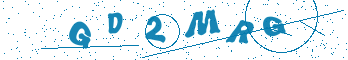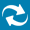# MathWorks MATLAB®

## Category : Co-Simulation Interfaces

Aldec simulators integrate The MathWorks' intuitive MATLAB language and a technical computing environment. It simplifies hardware design verification by providing robust visualization and analysis tools. It extends HDL testbenches with MATLAB language parts in order to create complex stimuli, perform UUT data analysis, or visualize simulated data as clearly as possible.You can execute MATLAB commands, call M-Function, or transfer data to or from the MATLAB workspace from within an level of HDL hierarchy. The Interface to MATLAB also provides users with the following benefits:

• Intuitive interface that fills the gap between HDL simulation and high-level mathematical computation
• Displaying simulation results with the use of robust set of graphical tools built-in the MATLAB environment.
• Automatic value conversion between Aldec simulators and MATLAB.
• Advanced testbenches employing complex mathematical formulas used to stimulate unit under test (UUT).

Related Application Note: Matlab – HDL Interface in Riviera-PRO and Active-HDL

Related Application Note: Controlling Riviera-PRO from MATLAB®